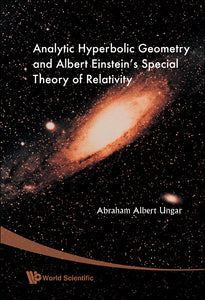# Analytic Hyperbolic Geometry And Albert Einstein's Special Theory Of Relativity

Abraham Albert Ungar

Format: Print Book

ISBN: 9789812772299

• SGD 254.66
Unit price per
Tax included.

This book presents a powerful way to study Einstein's special theory of relativity and its underlying hyperbolic geometry in which analogies with classical results form the right tool. It introduces the notion of vectors into analytic hyperbolic geometry, where they are called gyrovectors.Newtonian velocity addition is the common vector addition, which is both commutative and associative. The resulting vector spaces, in turn, form the algebraic setting for the standard model of Euclidean geometry. In full analogy, Einsteinian velocity addition is a gyrovector addition, which is both gyrocommutative and gyroassociative. The resulting gyrovector spaces, in turn, form the algebraic setting for the Beltrami-Klein ball model of the hyperbolic geometry of Bolyai and Lobachevsky. Similarly, Möbius addition gives rise to gyrovector spaces that form the algebraic setting for the Poincaré ball model of hyperbolic geometry.In full analogy with classical results, the book presents a novel relativistic interpretation of stellar aberration in terms of relativistic gyrotrigonometry and gyrovector addition. Furthermore, the book presents, for the first time, the relativistic center of mass of an isolated system of noninteracting particles that coincided at some initial time t = 0. The novel relativistic resultant mass of the system, concentrated at the relativistic center of mass, dictates the validity of the dark matter and the dark energy that were introduced by cosmologists as ad hoc postulates to explain cosmological observations about missing gravitational force and late-time cosmic accelerated expansion.The discovery of the relativistic center of mass in this book thus demonstrates once again the usefulness of the study of Einstein's special theory of relativity in terms of its underlying analytic hyperbolic geometry.

Format: Hardcover
No of Pages: 648
Imprint: World Scientific
Publication date: 20080213